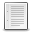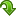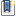File : Details

# cummin(), cummax()

Cumulative minima and maxima of array's values
Details
Version
1.0
Author
Samuel Gougeon
Maintainer
Samuel Gougeon
Categories
Supported Scilab Version
5.0
Creation Date
May 25, 2015Description
```            cummin(..) and cummax(..) functions

-->cummax
function [R] = cummax(A,orien,fromlast)

Cumulative maxima of values in a vector, matrix or hypermatrix

CALLING SEQUENCES
cummax           // displays this help
cummax demo ;    // runs a randomized demo
R = cummax(A)
R = cummax(A, orien)
R = cummax(A, orien, fromlast)

PARAMETERS
A, R : vector, matrix or hypermatrix of decimals, complex numbers, encoded
integers, or booleans. R gets the sizes and the type of A.

orien: 'r' | 1 | 'c' | 2 | n>2: the index of the dimension across which A
must be processed. 'r' or 1 means across rows ; 'c' or 2 means
across columns ; if A is an hypermatrix  with N dimensions, any
integer N>= n > 2 means across the dimension #n. Default value =1

fromlast: optional scalar boolean (default %F). If it is true, the processing
starts from the last element across the chosen orientation, instead
of starting from the first one.

DESCRIPTION
With R = cummax(A) or R = cummax(A,1) or R = cummax(A,"r"):
For all columns, pages etc, the line #i of R takes the maximal value met
across lines #1 to #i of A.
If the option fromlast is %T, the line #i of R takes the maximal value met
across lines #i to #\$ of A (where \$ stands for the index of the last line).
If A is boolean, %T is considered as 1 and %F is considered as 0.
If A contains complex numbers, numbers with maximal moduli are retained ;
in case of equal moduli, numbers with maximal phases are retained.

With R = cummax(A, 'c') or R = cummax(A, 2): A is processed accros columns:
For all lines, pages, etc, the column #i of R takes the maximal value met
across columns #1 to #i of A.

EXAMPLES
--------
// DECIMAL NUMBERS
-->a = grand(3,5,"uin",0,4)
a  = [
0.    0.    1.    2.    3.
4.    1.    0.    3.    2.
0.    4.    3.    2.    0.
]
-->cummax(a)
ans  =
0.    0.    1.    2.    3.
4.    1.    1.    3.    3.
4.    4.    3.    3.    3.

-->cummax(a,2, fromlast=%t)
ans  =
3.    3.    3.    3.    3.
4.    3.    3.    3.    2.
4.    4.    3.    2.    0.

// ENCODED INTEGERS
-->a = int8(a)
a  =
0  0  1  2  3
4  1  0  3  2
0  4  3  2  0

-->cummax(a,1, fromlast=%t)
ans  =
4  4  3  3  3
4  4  3  3  2
0  4  3  2  0

// BOOLEANS
-->a = a>2
a  =
F F F F T
T F F T F
F T T F F

-->cummax(a)
ans  =
F F F F T
T F F T T
T T T T T

// COMPLEX NUMBERS
-->c = grand(3,4,"uin",-2,2) + %i*grand(3,4,"uin",-2,2)
c  =
1. + i      1. - i      1. + i      i
1. + i      0           1. + 2.i    1. + 2.i
1. - i    - 2.        - 1. + i    - 2. - i

-->phases = atan(imag(c),real(c))
phases  =
0.7853982  - 0.7853982    0.7853982    1.5707963
0.7853982    0.           1.1071487    1.1071487
- 0.7853982    3.1415927    2.3561945  - 2.677945

-->cummax(c)
ans  =
1. + i      1. - i      1. + i      i
1. + i      1. - i      1. + 2.i    1. + 2.i
1. + i    - 2.          1. + 2.i    1. + 2.i

// HYPERMATRIX:
-->a = int8(grand(2,4,3,"uin",0,4))
a  =
(:,:,1)
3  1  2  3
1  2  2  2
(:,:,2)
4  1  0  3
1  3  1  1
(:,:,3)
0  4  2  0
4  4  0  3

-->cummax(a,3)
ans  =
(:,:,1)
3  1  2  3
1  2  2  2
(:,:,2)
4  1  2  3
1  3  2  2
(:,:,3)
4  4  2  3
4  4  2  3

// ===========================================================

-->cummin
function [R] = cummin(A,orien,fromlast)

Cumulative minima of values in a vector, matrix or hypermatrix

CALLING SEQUENCES
cummin           // displays this help
cummin demo ;    // runs a randomized demo
R = cummin(A)
R = cummin(A, orien)
R = cummin(A, orien, fromlast)

PARAMETERS
A, R : vector, matrix or hypermatrix of decimals, complex numbers, encoded
integers, or booleans. R gets the sizes and the type of A.

orien: 'r' | 1 | 'c' | 2 | n>2: the index of the dimension across which A
must be processed. 'r' or 1 means across rows ; 'c' or 2 means
across columns ; if A is an hypermatrix  with N dimensions, any
integer N>= n > 2 means across the dimension #n. Default value =1

fromlast: optional scalar boolean (default %F). If it is true, the processing
starts from the last element across the chosen orientation, instead
of starting from the first one.

DESCRIPTION
With R = cummin(A) or R = cummin(A,1) or R = cummin(A, 'r'):
For all columns, pages etc, the line #i of R takes the minimal value met
across lines #1 to #i of A.
If the option fromlast is %T, the line #i of R takes the minimal value met
across lines #i to #\$ of A (where \$ stands for the index of the last line).
If A is boolean, %T is considered as 1 and %F is considered as 0.
If A contains complex numbers, numbers with minimal moduli are retained ;
in case of equal moduli, numbers with minimal phases are retained.

With R = cummin(A, 'c') or R = cummin(A, 2): A is processed accros columns:
For all lines, pages, etc, the column #i of R takes the minimal value met
across columns #1 to #i of A.

EXAMPLES
--------
-->a = int8(grand(3,4,"uin",0,5))
a  = [
4  3  5  2
2  1  4  4
1  0  5  3
]
-->cummin(a)
ans  =
4  3  5  2
2  1  4  2
1  0  4  2

-->cummin(a, 2)
ans  =
4  3  3  2
2  1  1  1
1  0  0  0

-->cummin(a, 2, fromlast=%t)
ans  =
2  2  2  2
1  1  4  4
0  0  3  3
```Files (1)Miscellaneous file
```Macro defining both cummin() and cummax() functions

* MAKE IT AVAILABLE in EVERY SESSION: Click there to see indications

* Get help: Enter "cummin" or "cummax" without parameters

```News (0)Comments (1)Comment from Man Jane -- August 4, 2020, 10:53:11 PM
```https://www.gmsocrates.online/
```Leave a comment
You must register and log in before leaving a comment.Email notifications
Send me email when this toolbox has changes, new files or a new release.